### Line-Search Methods

In each iteration, the (dual) quasi-Newton, hybrid quasi-Newton, conjugate gradient, and Newton-Raphson minimization techniques use iterative line-search algorithms that try to optimize a linear, quadratic, or cubic approximation of
along a feasible descent search direction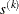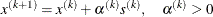by computing an approximately optimal scalar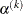.

Therefore, a line-search algorithm is an iterative process that optimizes a nonlinear function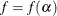of one parameter () within each iterationof the optimization technique, which itself tries to optimize a linear or quadratic approximation of the nonlinear objective function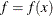ofparameters. Since the outside iteration process is based only on the approximation of the objective function, the inside iteration of the line-search algorithm does not have to be perfect. Usually, the choice ofsignificantly reduces (in a minimization) the objective function. Criteria often used for termination of line-search algorithms are the Goldstein conditions (refer to Fletcher (1987)).

Various line-search algorithms can be selected using the LINESEARCH= option. The line-search method LINESEARCH=2 seems to be superior when function evaluation consumes significantly less computation time than gradient evaluation. Therefore, LINESEARCH=2 is the default value for Newton-Raphson, (dual) quasi-Newton, and conjugate gradient optimizations.

A special default line-search algorithm for TECH=HYQUAN is useful only for least squares problems and cannot be chosen by the LINESEARCH= option. This method uses three columns of theJacobian matrix, which for largecan require more memory than using the algorithms designated by LINESEARCH=1 through LINESEARCH=8.

The line-search methods LINESEARCH=2 and LINESEARCH=3 can be modified to exact line search by using the LSPRECISION= option (specifying theparameter in Fletcher (1987)). The line-search methods LINESEARCH=1, LINESEARCH=2, and LINESEARCH=3 satisfy the left-hand-side and right-hand-side Goldstein conditions (refer to Fletcher (1987)). When derivatives are available, the line-search methods LINESEARCH=6, LINESEARCH=7, and LINESEARCH=8 try to satisfy the right-hand-side Goldstein condition; if derivatives are not available, these line-search algorithms use only function calls.